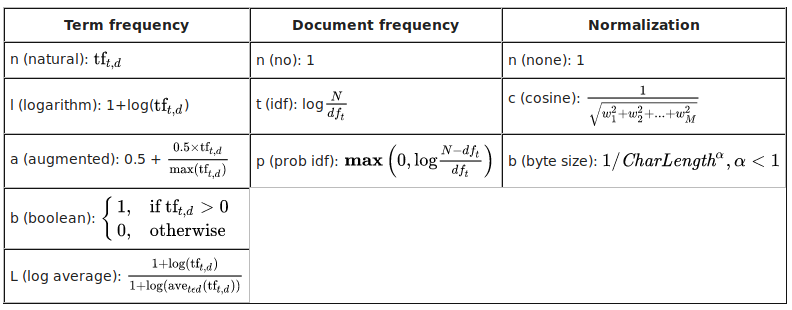• ## TFIDF

2019-09-14 16:25:20
目录1.TFIDF算法原理2. TFIDF 概率模型解释3. TFIDF python 实战 1.TFIDF算法原理 TFIDF (term frequency - inverse document frequency) 主要思想：如果某个词或者短语在一篇文章中出现的频率TF较高，而且在其他...
目录1.TFIDF算法原理2. TFIDF 概率模型解释3. TFIDF python 实战

1.TFIDF算法原理
TFIDF (term frequency - inverse document frequency)
主要思想：如果某个词或者短语在一篇文章中出现的频率TF较高，而且在其他文章中出现的频率较少，则认为此词或短语具有很好的类别区分能力。
计算公式：
有语料库$D$，文章表示为 $d$，文章中的词或者短语表示为$w$。
TF(term frequency，词频):
$TF_{w, d_i} = \frac{count(w)} {\sum\limits_{t \in d_i} count(t)}$
$count(w)$为关键词$w$出现的次数。
IDF(inverse document frequency, 逆文档频率):
$IDF_{w, D} = log \frac{|D|} {1 + \sum\limits_{i = 1}^{|D|} I(w, d_i) }$
$|D|$表示语料库$D$中文章的数量，$I(w, d_i)$为指示函数，表示词或者短语$w$是否在文章$d_i$中出现，出现为1，否则为0。
若词或者短语$w$未在语料库中出现过，我们需要对$IDF$进行平滑(smooth)操作。
$TFIDF_{w, d_i} = TF_{w, d_i} * IDF_{w, D}$

一些结论：

当一个词在文章频率越高并且新鲜度高（即普遍度低），其TF-IDF值越高。
TF-IDF兼顾词频与新鲜度，过滤一些常见词，保留能提供更多信息的重要词。

2. TFIDF 概率模型解释

参考网页

3. TFIDF python 实战

参考网页


展开全文算法
• ## tfidf

2020-05-25 14:46:58
tfidf包括两部分：tf：词语在文档中出现的次数，idf中的df是在整个语料库中有多少篇文档包含了该词语，其中的i是逆的意思，核心思想是：如果一个词在某篇文档中出现的次数多并且包含该词语的文档少，那么这个词语很...
tfidf包括两部分：tf：词语在文档中出现的次数，idf中的df是在整个语料库中有多少篇文档包含了该词语，其中的i是逆的意思，核心思想是：如果一个词在某篇文档中出现的次数多并且包含该词语的文档少，那么这个词语很重要。如果一个词在很多个文档中都出现，那么说明这个词只是个高频词，没有意义（例如：“的”这个字）。

tfidf有很多变种，可以参考下面图表。上图的出处：https://rare-technologies.com/pivoted-document-length-normalisation/

gensim中的函数是通过指定字母来确定用哪个公式，

上面是理论方面，实现方面，可以用gensim也可以用sklearn

额外要补充的：

1.gensim里面可以自定义wlocals（也就是tf的函数）和wglobal（也就是idf的函数）

2.gensim里面有pivot和slope，据说可以解决cosine normalize倾向于给短文本赋予更大权重的问题。

slope * old_norm + (1.0 -slope) * pivot

这两个参数有默认值，默认值就挺好的，基本不用动，pivot是如果有corpus或者dictionary的话，会自动设置，而slope是取的一个经典的值0.25。

展开全文• ## Tfidf

2016-06-13 15:59:40
Tfidf(词频-反转文档频率）TF代表统计部分， 而IDF把权重折扣考虑了进去。>>> import scipy as sp >>> def tfidf(term, doc, docset): ··· tf = float(doc.count(term) / sum(doc.count(term) for doc in docset)...
Tfidf(词频-反转文档频率）

TF代表统计部分， 而IDF把权重折扣考虑了进去。

>>> import scipy as sp
>>> def tfidf(term, doc, docset):
···     tf = float(doc.count(term) / sum(doc.count(term) for doc in docset)
···     idf = math.log(float(len(docset)) / (len([doc for doc in docset
if term in doc]))
···     return tf * idf
展开全文• <p>I recently used dask to implement a distributed version of tfidf. I want to contribute to the dask project by putting it somewhere. <p>Would this be the correct repo.? <p>I thought maybe a <code>...
• Tfidf计算 主要内容 读取全部评论与词频构成的xls文档 读取对评论初步分词后的文档和停用词txt文档 将每条评论的词汇与词频输出为字典形式，同时每条评论整体输出为一个列表元素（方便后续构造稀疏矩阵） 计算各...
Tfidf计算

主要内容

读取全部评论与词频构成的xls文档
读取对评论初步分词后的文档和停用词txt文档
将每条评论的词汇与词频输出为字典形式，同时每条评论整体输出为一个列表元素（方便后续构造稀疏矩阵）
计算各词语的tf-idf值并输出到xls文档

具体实现
读取评论文档，并分词、统计词频
将分词后的词语与频数输出为字典格式

import xlrd
import thulac
import xlwt
import numpy as np
from sklearn.feature_extraction import DictVectorizer

data = xlrd.open_workbook('E:\\wholeComm_wordCount_book1_570.xls')
table = data.sheet_by_name('wholeComm_wordCount_book1_570')

table1 = table.col_values(0)

# 对评论文档进行分词
thu1 = thulac.thulac(seg_only = True)
thu1.cut_f("E:\\wholeComm_book01_570.txt", "E:\\wholeComm_book01_570cutput2.txt")

file = open("E:\\wholeComm_book01_570cutput2.txt", 'r')   # 待统计文档的路径
file2 = open("E:\\Octopus\\task01_book\\StopWords.txt", 'r', encoding='utf-8')    # 待统计文档的路径

# 将每条评论的词汇与词频输出为字典形式，同时每条评论整体输出为一个列表元素
vector = []
for line in txt:
txtline = str(line)
lineword = txtline.split(' ')   # 将文本按照空格切分，获得列表

count = 0
dict1 = {}
for word in lineword[:-1]:
if word in stopwords:   # 不统计停用词
pass
else:
if word in dict1:
count += 1
dict1[word] = count
else:
# print("A word lost at sum.txt:", word)
count = 1
dict1[word] = count
vector.append(dict1)

file.close()
file2.close()

计算tf-idf值
#  CountVectorizer函数，属于常见的特征数值计算类，是一个文本特征提取方法。
#  对于每一个训练文本，它只考虑每种词汇在该训练文本中出现的频率，将文本中的词语转换为词频矩阵。
vecorizer = DictVectorizer(sparse=False)    # 设置sparse=False获得numpy ndarray形式的结果
matrix = vecorizer.fit_transform(vector)    # 对字典列表vector进行转换，转换成特征矩阵(分词后，所有词汇形成的稀疏矩阵)

sumword = [sum(i) for i in zip(*matrix)]    # 计算一列的总值：各词在全部评论中出现的总次数，矩阵长度为词的类别数
transpose = list(map(list, zip(*matrix)))   # 将矩阵转置，用以统计含有特定词的评论数
sentence = [len(i)-i.count(0) for i in transpose]   # 含有对应词语的评论数
tf = [round(i/sum(sumword),4) for i in sumword]
idf = [round(np.log(len(matrix)/(i+1)),4) for i in sentence]
tfidf = [round(tf*idf,4) for tf,idf in zip(tf,idf)]

sort_tfidf = list(np.sort(tfidf))      # 将各词语的tfidf数值按照升序排列
index = list(np.argsort(sentence))      # 升序排列的词在原列表中的索引
rank = [[sort_tfidf[-i] for i in range(1,81)], [index[-i] for i in range(1,81)]]    # 输出前81个tfidf值和其在原序列中的索引

wordname = vecorizer.get_feature_names()    # 获取字典的键：文本中的词
feature = [wordname[i] for i in rank]    # 获取tfidf值排在前81的词
print('wordname:', wordname)
print('feature:', feature)

wbk3 = xlwt.Workbook(encoding='utf8')
for i in range(len(feature)):
sheet.write(i, 1, label=tfidf[i])
sheet.write(i, 0, label=feature[i])
wbk3.save('E:\\wholeComm_word_80tfidf.xls')




展开全文自然语言处理
• tfidf java实现 适用于单篇文档提取关键词 实测效果还行
• class TFIDF(object):"""以一个图书馆为例，tf: 该单词在图书馆某本书里出现的频率idf: 1+log((图书馆所有书的数量+平滑系数)/(该单词出现过的书的数量+平滑系数))tfidf = tf*idf，即对应该本书该词的tfidf值"""def ...
• 简单实现tfidf算法，tfidf算法大家众所周知，就是用来计算词的权重的
• lucene tfidf score获取 idf indexReader.docFreq(new Term(FIELD, “中国”)) indexReader.maxDoc() tf Terms terms = indexReader.getTermVector(docID, TEXT_FIELD); TermsEnum termsEnum = terms.iter.....
• function [count,tf,idf,weight]=tfidf(docs,term)%docs--input documents，cell型%term-- keywords也就是特征词提取,cell型%output:count--存放各个关键词出现的频率在整个文档中% wordnum--存放文档总的词汇数%...
• 代码对10个txt文件进行分词、去除停止词，并提取每个词的tfidf特征值输出
• TFIDF是经典的算法，可以进行文本相似度计算和文档聚类，值得研究
• tfidf的python实现，用语文本分类时的特征提取，非常实用，
• 利用java实现TFIDF，提取关键词，是术语提取、推荐系统等应用的基础之一。
• VSM TFIDF 空间向量模型 Adapted from Lectures by Prabhakar Raghavan (Yahoo and Stanford) and Christopher Manning (Stanford)
• 司法领域关键词及其tfidf值，主要面向罪名预测研究方向，涉及100多种罪名文本类型。当然，在司法领域的其他研究方向上，也有一定的参考价值。
• 读取82个txt文件，文本预处理，计算tfidf值，将矩阵导出excel，获取词袋，将词袋写入excel，计算余弦相似度并导出excel
• 文档介绍了tfidf算法的由来，对权重计算做了详细介绍，对tfidf的发展做了个综述
• 基于MR实现的TFIDF相似度的计算过程，可以深刻理解mapreducer的过程同时了解TFIDF计算过程...